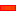﻿ Degrees-of-Freedom and Mobility

# Degrees-of-Freedom and Mobility

### Degrees-of-Freedom and Mobility

This topic reviews:

 • Degrees-of-Freedom on Parts and Joints
 • Degrees-of-Freedom of a Kinematic-Chain and the Gruebler Equation
 • Mobility of kinematic-chains
 • Degrees-of-Freedom of Open and Closed kinematic-chains

####Degrees-of-Freedom and PartsImage from SOLIDWORKS

DOF of a Part [General]

Parts free to move in space have six degrees-of-freedom [D.O.F.].

Why six? Take the image to the left, you can see that a Part, in general 'Global Coordinates:

 • can 'translate' [move linearly] along the three axis directions and coordinates: X, Y, Z
 • can 'rotate' about the three linear axes, say with rotation coordinates: α, β, γDOF of a Free-Part on a Mechanism-Plane

In MechDesigner, Parts lie on a Mechanism-Plane.

A Free-Part has three D.O.F.

The Part can translate along the: x and y directions.

The Part can rotate about axis that is normal to the Mechanism-Plane: θ

####Degrees-of-Freedom removed by a single JointPin-Joint: Requires a Line in the moving Part to remain co-linear with a Line in the Mechanism-Plane, and a plane perpendicular to this Line in the moving Part maintain contact with a similar perpendicular plane in the Mechanism-Plane. A Pin-Joint removes two degrees-of-freedom - the Part has one degree-of-freedom remaining - it can rotate. Slide-Joint Requires that a Line in the moving Part remains co-linear with a line in the Mechanism-Plane, and a plane parallel to this Line in the moving Part maintain contact with a similar parallel plane in the Mechanism-Plane. A Slide-Joint removes two degrees-of-freedom - the Part has one degree-of-freedom remaining - it can slide.

####DOF and Gruebler Equation

 DOF of a Kinematic-Chain? Kinematic-chains may have many Parts and Joints. Fortunately, we can use the Gruebler Equation to calculate the number of degrees-of-freedom of most kinematic-chains. Gruebler Equation For a Planar kinematic-chain, the Gruebler Equation is: F = 3*(N-1) – 2*J – H MechDesigner automatically calculates the number of Degrees-of-Freedom in a Kinematic-Chain. Note: if the Degrees-of-Freedom = Zero, then the kinematic-chain is a structure. where: F = degrees-of-freedom for the kinematic-chain N = number of parts - include the Base-Part J = number of one degree-of-freedom joints H = number of 'higher-pair' joints - see Joints It is important to notice that the Gruebler Equation does not need to know the lengths or shape of parts or the type of joint.

####Mobility of a Kinematic-ChainMOBILITY = Number of Motion Dimensions – Number of Degrees-of-Freedom When the Mobility = 0  the Kinematic-Chain is a kinematically-defined chain. The Target: Number of Motion-Dimension FBs = Number of D.O.F. in the Kinematic-Chain. The Kutzbach Criterion states that for a kinematic-chain to be useful, the number of 'drives' (motion-dimension) must equal the number of degrees-of-freedom.

####Degrees-of-Freedom and Mobility of 'Open' and 'Closed' Kinematic-Chains

Degrees-of-Freedom and Mobility: without Motion Dimensions

####Open Kinematic ChainThe image shows an 'open' kinematic-chain. There are four Parts (the Base-Part + 3 Added-Parts), with three Joints. Gruebler Equation: F = 3* (4-1) – 2*3 – 0 = 3 . Three degrees-of-freedom. Mobility =  Number of Motion Dimensions - Number of Degrees-of-Freedom Mobility = 0 – 3 = -3

####Closed Kinematic ChainThe image shows a 'closed' kinematic-chain. There are still four Parts, but now with four Joints. Gruebler Equation: F = 3* (4-1) – 2*4 – 0 = 1. One Degree-of-Freedom Mobility =  Number of Motion Dimensions - Number of Degrees-of-Freedom Mobility = 0 – 1 = -1

Degrees-of-Freedom and Mobility: with Motion Dimensions

####Open Kinematic ChainAdd three Motion-Dimension FBs. It had three Degrees-of-freedom. Mobility =  Number of Motion Dimensions - Number of Degrees-of-Freedom Mobility = 3 – 3 = 0

####Closed Kinematic ChainAdd on Motion Dimension to the kinematic-chain It had one Degree-of-freedom, . Mobility = Number of Motion Dimensions - Number of Degrees-of-Freedom Mobility = 1 – 1 = 0

Tutorial and Reference Help Files for MechDesigner and MotionDesigner 13.2 + © Machine, Mechanism, Motion and Cam Design Software by PSMotion Ltd